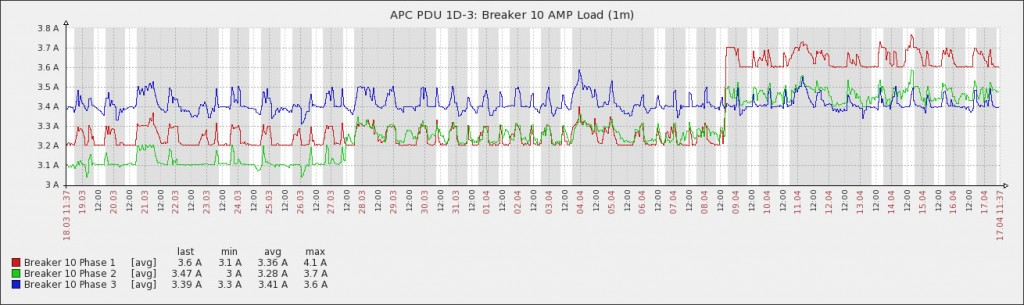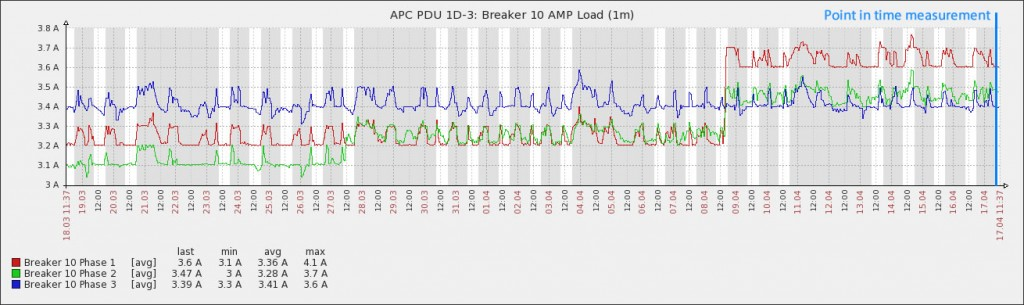# Utility Billing – Explained

In Part III of How Enseva Makes Colocation Better, I touched briefly on the topic of how kilowatt-hour usage calculations will often vary between colocation providers. As promised, I plan to elaborate and compare the different methodologies I’ve personally seen in various multitenant facilities.

POINT-IN-TIME
The most common method I see utilized requires a technician with a mobile Current Clamp or Current Probe designed to non-invasively measure the volume of power consumed using a current transformer. The clap is attached around the electrical wires (usually in a breaker distribution panel) and provides a real-time measurement in amperes. These ampere measurements, or “amps,” are later used in a formula to estimate the amount of power consumed.

Below is an electrical graph generated by Pentagon (Enseva’s monitoring platform). In this graph, we see three individual lines. Each line represents a phase of power in a three-phase receptacle. This particular breaker happens to be an L21-30 (208v 3P 30Amp) breaker. The resolution of the graph is 30 days, and we can clearly see not only fluctuations in electrical draw throughout the day, but also several distinct jumps in electrical usage consistent with new servers being deployed.At the end of the month, a datacenter technician would use a current prove to lease the amount of current consumed by the particular electrical breaker supplying power to a cabinet. In the graph below, the Enseva Blue line to the far right represents the point when that measurement would have been taken by the technician.The results of the measurements would have been 3.65A for Phase I, 3.5A for Phase II and 3.4A for Phase III.  Because we happen to be measuring individual 120v delivered, the math to convert amps to Watts/kWatts is simple.  Amps * Volts / 1000 = kilowatts.

3.65 * 120v / 1000 = 0.438kW

3.65 * 120v / 1000 = 0 .42kW

3.65 * 120v / 1000 = 0.408kW

Which — when added up — provides us with a total of 1.266kW of current load. This happens to be a rather lightly loaded cabinet compared to others throughout the facility. Regardless of the electrical draw, a very valid argument holds true.

We now need to add a volume of time to the equation. Kilowatt-hour is the measure of a unity of energy over a period of one hour and is the standard measurement used by utility companies to bill for consumed electricity. For the purposes of this blog, we’ll assume the present month has 30 days. So converting a 1.266kW load to kilowatt-hours is as simple as kW * 24hrs * 30Days or 911.52kWh’s.

The datacenter would then invoice the customer at whatever its current electrical rate is times the 911.52 value it just came up with. If you haven’t already noticed, the previous graphs show a technician taking usage measurements at the tail end of a month, at the peak of that particular customer’s energy usage. You may be asking, “Does this mean the customer paid for energy that wasn’t actually consumed at the beginning of the month?” The answer is “Yes!”

The measurement resolution for these point-in-time readings is one month. This means that whenever the measurement was taken, it’s assumed to be your average usage and that average usage is the basis for determining your electrical bill for the entire month. We can clearly see that in this case that’s not a true statement.

REAL TIME
Thanks to Enseva’s branch-circuit monitoring systems, measurements are taken in five-minute samples, which gives us a resolution of five minutes as opposed to one month. This level of resolution permits Enseva to capture intricate details on customer loads, which you can see in the previous graphs.

When we reviewed the same breaker above, during the exact same time frame, we found that the actual kWh usage for this circuit was 780kWh’s when using the higher resolution measurements. The difference between the two methods is approximately 132kWh’s, or 14.4%. When multiplied by hundreds of breakers, this could result in tens of thousands of dollars in savings annually, in addition to the other benefits outlined in the article this blog post is referencing.